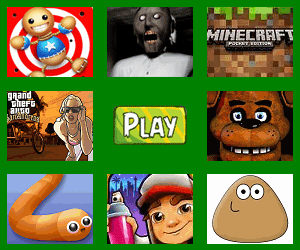﻿ 使用 Win2D 實現融合效果_ZenDei技術網路在線

# 使用 Win2D 實現融合效果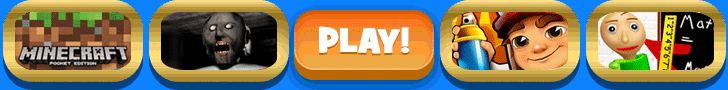1. 融合效果 在 CSS 中有一種實現融合效果的技巧，使用模糊濾鏡（blur）疊加對比度濾鏡（contrast）使兩個接近的元素看上去“粘”在一起，如下圖所示： 博客園的 ChokCoco 就用這個技巧實現了很多不同的玩法並寫了很多文章，例如這篇： 你所不知道的 CSS 濾鏡技巧與細節 我一直對這 ...

## 1. 融合效果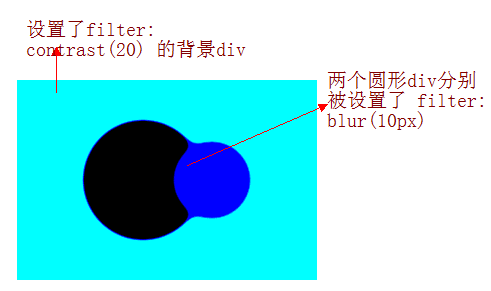## 2. 使用 Win2D 實現融合效果

Win2D 是一個很簡單使用的底層圖形 Windows Runtime API。它利用了 Direct2D 的強大功能，並與 UWP 和 WinUI 3 的 Xaml 無縫集成，可以使用強大的渲染得到漂亮界面。UWP 和 WinUI 3 版本的 Nuget 安裝腳本分別為：

Install-Package Win2D.uwp -Version 1.26.0

Install-Package Microsoft.Graphics.Win2D -Version 1.0.3.1

``````private ICanvasBrush _leftBrush;
private ICanvasBrush _rightBrush;
private ICanvasImage _image;
private GaussianBlurEffect _blurEffect;

private void OnCreateResource(CanvasControl sender, CanvasCreateResourcesEventArgs args)
{
_leftBrush = new CanvasSolidColorBrush(sender, Colors.Black);
_rightBrush = new CanvasSolidColorBrush(sender, Colors.Blue);
_blurEffect = new GaussianBlurEffect
{
BlurAmount = 20f
};

_image = new ColorMatrixEffect
{
ColorMatrix = new Matrix5x4
{
M11 = 1,
M12 = 0,
M13 = 0,
M14 = 0,
M21 = 0,
M22 = 1,
M23 = 0,
M24 = 0,
M31 = 0,
M32 = 0,
M33 = 1,
M34 = 0,
M41 = 0,
M42 = 0,
M43 = 0,
M44 = 18,
M51 = 0,
M52 = 0,
M53 = 0,
M54 = -7
},
Source = _blurEffect
};
}
``````

``````private void OnCanvasSizeChanged(object sender, SizeChangedEventArgs e)
{
_centerPoint = Canvas.ActualSize / 2;
}
``````

``````private void OnDraw(CanvasControl sender, CanvasDrawEventArgs args)
{
var source = new CanvasCommandList(sender);
using (var drawingSession = source.CreateDrawingSession())
{
drawingSession.FillCircle(_centerPoint + new Vector2(-75, 0), 100, _leftBrush);
drawingSession.FillCircle(_centerPoint + new Vector2(75, 0), 60, _rightBrush);
}

_blurEffect.Source = source;
args.DrawingSession.DrawImage(_image);
}
``````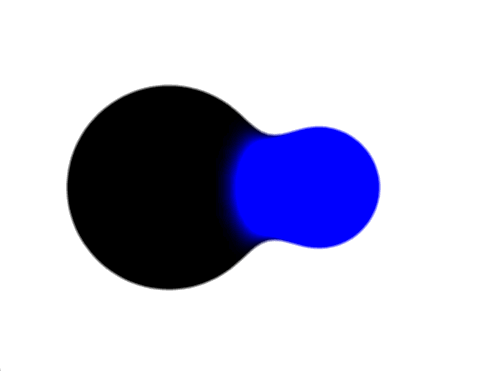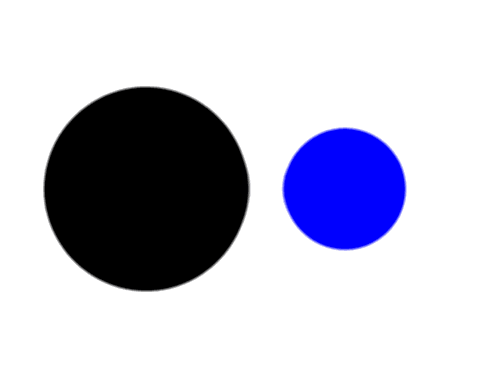## 3. Win2D 中融合效果的原理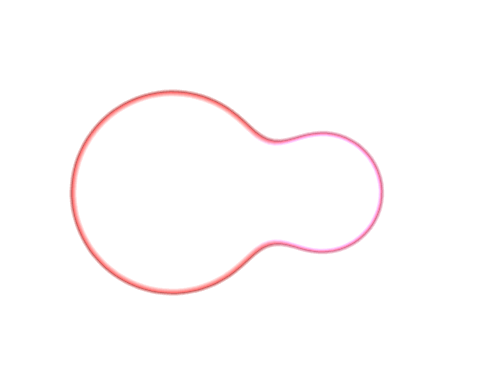### 3.1 GaussianBlurEffect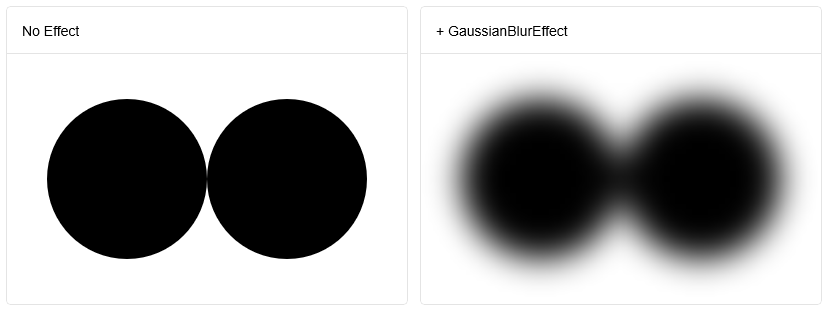### 3.2 ColorMatrixEffect

ColorMatrixEffect 使用 Matrix5x4 修改圖像的顏色。這個 Matrix5x4 的預設值是：

``````var identity = new Matrix5x4
{
M11 = 1, M12 = 0, M13 = 0, M14 = 0,
M21 = 0, M22 = 1, M23 = 0, M24 = 0,
M31 = 0, M32 = 0, M33 = 1, M34 = 0,
M41 = 0, M42 = 0, M43 = 0, M44 = 1,
M51 = 0, M52 = 0, M53 = 0, M54 = 0
};
``````

``````result.R = (src.R * matrix.M11) + (src.G * matrix.M21) + (src.B * matrix.M31) + (src.A * matrix.M41) + matrix.M51
result.G = (src.R * matrix.M12) + (src.G * matrix.M22) + (src.B * matrix.M32) + (src.A * matrix.M42) + matrix.M52
result.B = (src.R * matrix.M13) + (src.G * matrix.M23) + (src.B * matrix.M33) + (src.A * matrix.M43) + matrix.M53
result.A = (src.R * matrix.M14) + (src.G * matrix.M24) + (src.B * matrix.M34) + (src.A * matrix.M44) + matrix.M54
``````

``````result.A =  (src.A * 18) -7
``````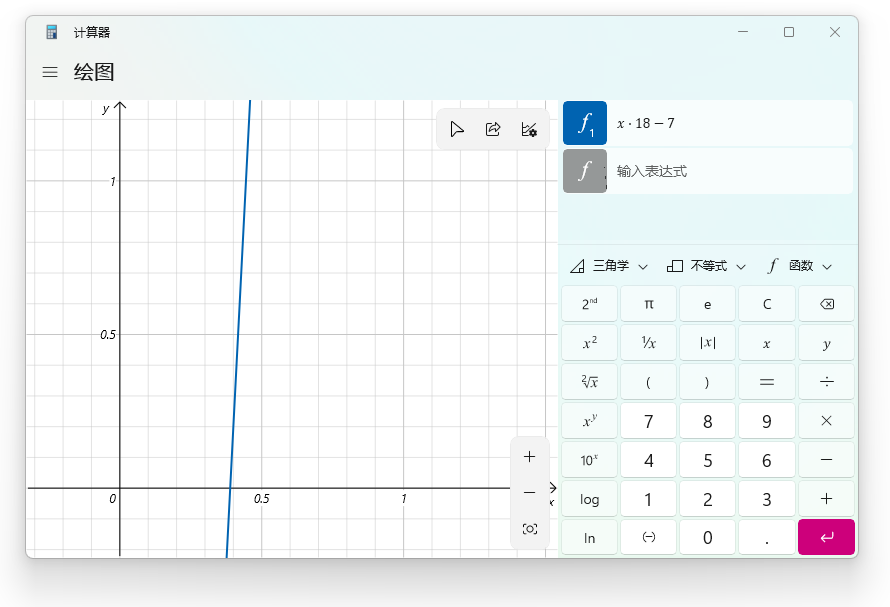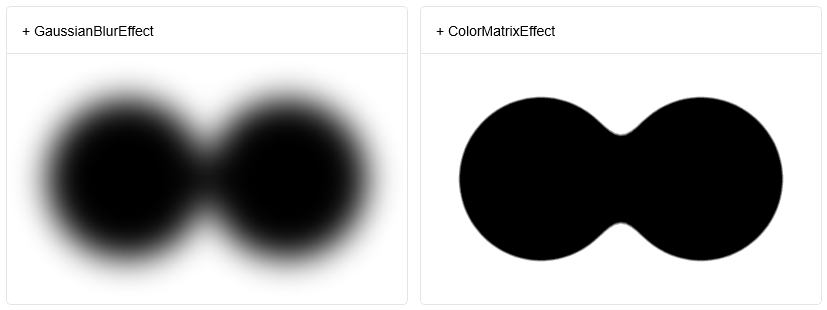### 3.3 ClampOutput

``````result.R = source.R * source.A;
result.G = source.G * source.A;
result.B = source.B * source.A;
result.A = source.A;
``````

Win2D: Premultiplied alpha

## 4. 最後## 6. 參考

cnbluefire_GooeyButton

https://github.com/Microsoft/Win2D

ColorMatrixEffect Class

GaussianBlurEffect Class• 框架介紹 1.1.1 概述 Dubbo是阿裡巴巴公司開源的一個高性能優秀的服務框架，使得應用可通過高性能的 RPC 實現服務的輸出和輸入功能，可以和 Spring框架無縫集成。 Dubbo是一款高性能、輕量級的開源Java RPC框架，它提供了三大核心能力：面向介面的遠程方法調用，智能容錯和負載均衡 ...
• 容器設計是項目編碼中非常常見的一個場景，本文從項目中習以為常的一些場景作為切入點，聊一聊容器設計的一些思考、聊一聊元素遍歷的思路、以及身為設計模式之一的迭代器模式。 ...
• 我的博客 一直有個想法，想要弄個微信機器人，然而出師不利，剛開始就碰壁了 先上代碼，這個是用來接收消息的，是個測試腳本 #!/usr/bin/python # coding: utf-8 import itchat def write_infomation(text_value): print(te ...
• WPS表格文件是金山開發的專門用於處理表格數據的Office工具，屬於WPS Office中WPS文字、WPS表格和WPS演示三大功能模塊之一。通常以.et和.ett作為文件尾碼。我們在通過後端來操作WPS表格文件時，可以通過以下方法來載入、編輯以及保存WPS表格文件，本文將對此做詳細介紹。 引入j ...
• 1 #include <stdio.h> 2 #include <malloc.h> 3 #include <stdlib.h> 4 #define MaxSize 50 5 #define InitSize 100 6 typedef int ElemType; 7 typedef struct ...
• 問題描述：新創建maven項目後，在父工程中dependencyManagement時，會報紅線錯誤，刷新後還是報紅，例如：\${spring.version}爆紅： Maven使用dependencyManagement元素來進行依賴版本的管理。具體來說，maven沿著父子層向上尋找，直到找到dep ...
• 在上一篇：UWP/WinUI3 PixelShaderEffect 實現ThresholdEffect 濾鏡。 - 吃飯/睡覺 - 博客園 (cnblogs.com) 已經價紹瞭如何編寫hsls，編譯，和使用 PixelShaderEffect 來實現自定義濾鏡效果了，那麼本編將介紹如何編寫一個 “ ...
• 在上一個文章中，傳送門，給大家介紹了怎麼在配置文件中使用 Kestrel 部署 Https，正好今天有小伙伴穩問到：可以通過代碼的方式實現 Kestrel 的 Https 的部署嗎？答案是肯定的，我們這次一樣去不是多個功能變數名稱。 在使用代碼實現中，我是主要使用到 ListenOptions.UseHtt ...